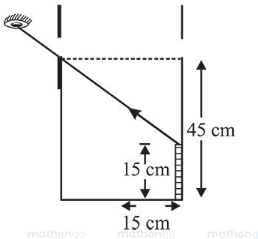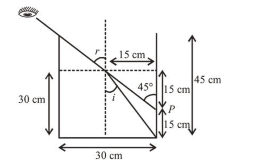# An observer can see through a small hole on the side of a jar (radius 15 cm )Question:

An observer can see through a small hole on the side of a jar (radius $15 \mathrm{~cm}$ ) at a point at height of $15 \mathrm{~cm}$ from the bottom (see figure). The hole is at a height of $45 \mathrm{~cm}$. When the jar is filled with a liquid up to a height of $30 \mathrm{~cm}$ the same observer can see the edge at the bottom of the jar. If the refractive index of the liquid is $N / 100$, where $N$ is an integer, the value of $N$ is_________

Solution:

(158)From figure, $\sin i=\frac{15}{\sqrt{15^{2}+30^{2}}}$ and $\sin r=\sin 45^{\circ}$

From Snell's law, $\mu \times \sin i=1 \times \sin r$

$\Rightarrow \mu \times \frac{15}{\sqrt{15^{2}+30^{2}}}=1 \times \sin 45^{\circ}=\frac{1}{\sqrt{2}}$$\therefore \mu=\frac{\frac{1}{\sqrt{2}}}{\frac{15}{\sqrt{1125}}}=158 \times 10^{-2}=\frac{N}{100}$

Hence, value of $N=158$.